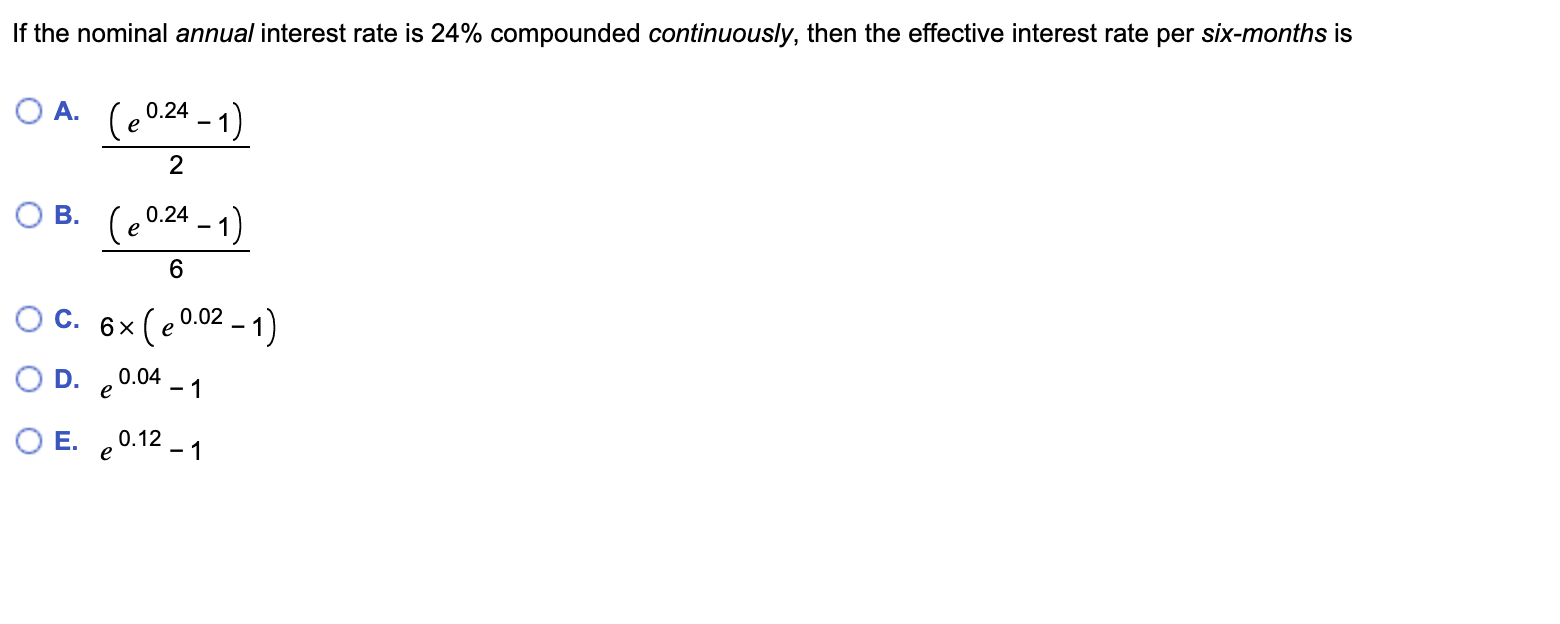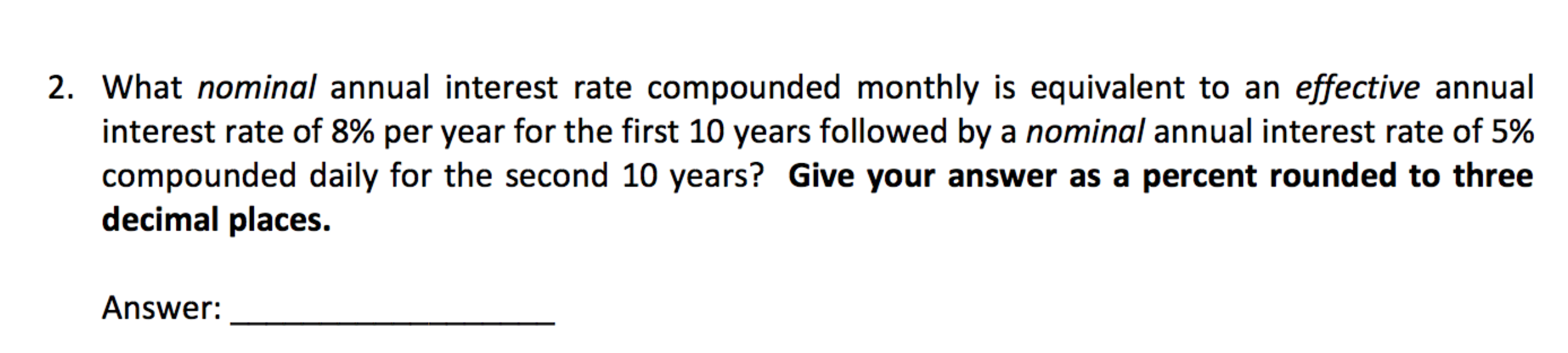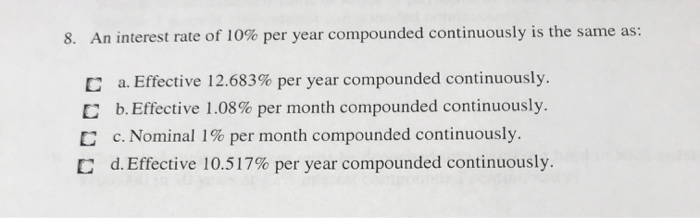Question

# What effective interest rate per two-months, compounded continuously, is equivalent to a nominal rate of 10%...

What effective interest rate per two-months, compounded continuously, is equivalent to a nominal rate of 10% per year?

The effective interest rate per two-months is _______ %

The formula for Effective interest rate when continuously compounding = ert - 1, where

r: rate per period = 10%/6= 0.01667 and

t: Number of period per year = 6

Effective interest rate with continuous compounding = e(0.01667 x 6) - 1 = e0.10002 - 1 = 0.10519 = 10.52%

#### Earn Coins

Coins can be redeemed for fabulous gifts.

Similar Homework Help Questions
• ### What effective interest rate per week, compounded continuously, is equivalent to a nominal rate of 10%...

What effective interest rate per week, compounded continuously, is equivalent to a nominal rate of 10% per year? The effective interest rate per week is ______%. It wasn't 10.52%, 10.517%, 10.506%, 10.51%, 10.37%.

• ### If the nominal annual interest rate is 24% compounded continuously, then the effective interest rate per...If the nominal annual interest rate is 24% compounded continuously, then the effective interest rate per six-months is OA. (20.24 - 1) OB. (20.24 - 1) O C. 6x (20.02 - 1) OD. €0.04 - 1 O E. 20.12 - 1

• ### What nominal rate per week is equivalent to an effective rate of 11% per year

What nominal rate per week is equivalent to an effective rate of 11% per year, compounded continuously? The nominal rate per week that is equivalent to an effective rate of 11% per year, compounded continuously is _______.

• ### 4. Find the effective bimonthly interest rate equivalent to: (a) nominal annual interest of 9%, compounded...

4. Find the effective bimonthly interest rate equivalent to: (a) nominal annual interest of 9%, compounded 6 times per year; (b) nominal annual discount of 6%, compounded quarterly; (c) 1/2 nominal annual interest of 8%, compounded continuously.

• ### 2. What nominal annual interest rate compounded monthly is equivalent to an effective annual interest rate...2. What nominal annual interest rate compounded monthly is equivalent to an effective annual interest rate of 8% per year for the first 10 years followed by a nominal annual interest rate of 5% compounded daily for the second 10 years? Give your answer as a percent rounded to three decimal places. Answer:

• ### What nominal interest rate compounded continuously is equivalent to j52 = 14%?

What nominal interest rate compounded continuously is equivalent to j52 = 14%?

• ### What nominal interest rate compounded continuously is equivalent to j52 = 14%?

What nominal interest rate compounded continuously is equivalent to j52 = 14%?

• ### 8? 8. An interest rate of 10% per year compounded continuously is the same as: C...8? 8. An interest rate of 10% per year compounded continuously is the same as: C D a. Effective 12.683% per year compounded continuously. b. Effective 1.08% per month compounded continuously. c. Nominal 1% per month compounded continuously. d. Effective 10.517% per year compounded continuously. C

• ### a. For an interest rate of 100% per year compounded continuously, calculate the effective daily, weekly,...

a. For an interest rate of 100% per year compounded continuously, calculate the effective daily, weekly, monthly, quarterly, semiannually, and annually interest rates. b. An investor requires an effective return of at least 12% per year. What is the minimum annual nominal rate that is acceptable for continuous compounding?

• ### What is the effective annual interest rate equivalent of a nominal rate given as 12% compounded...

What is the effective annual interest rate equivalent of a nominal rate given as 12% compounded quarterly?